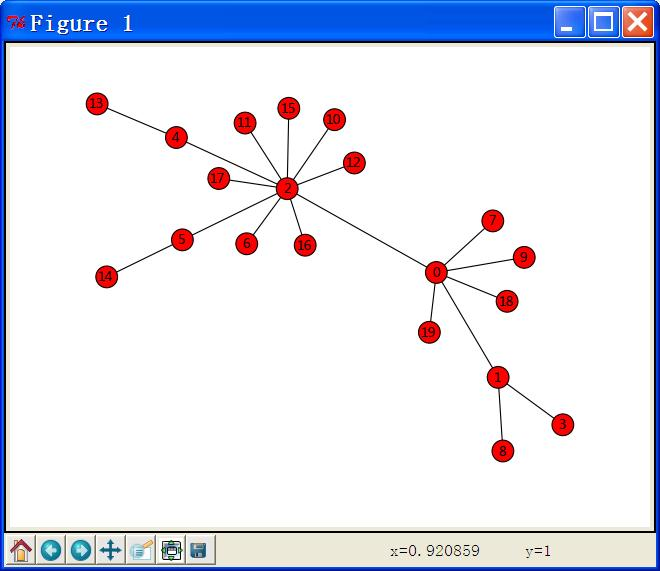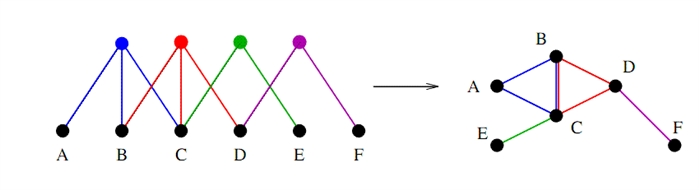# networkx笔记

1、无向图

import networkx as nx                            #导入NetworkX包，为了少打几个字母，将其重命名为nx
G = nx.Graph()                                        #建立一个空的无向图G
print G.nodes()                                       #输出全部的节点： [1, 2, 3]
print G.edges()                                       #输出全部的边：[(2, 3)]
print G.number_of_edges()                    #输出边的数量：1

2、有向图

3、加权图（网络）

print G.get_edge_data(1,2)                   #输出{'weight': 7.5}，这是一个字典结构，可以查看python语法了解它的用法。

NetworkX提供了常用的图论经典算法，例如DFS、BFS、最短路、最小生成树、最大流等等，非常丰富，如果不做复杂网络，只作图论方面的工作，也可以应用NetworkX作为基本的开发包。具体的算法调用方法我就不一一介绍了，可以浏览NX的在线手册http://networkx.lanl.gov/reference/algorithms.html，对每个算法都提供了详细的帮助文档和示例。下面只给出一个最短路算法的例子：
path=nx.all_pairs_shortest_path(G)     #调用多源最短路径算法，计算图G所有节点间的最短路径
print path                                     #输出节点0、2之间的最短路径序列： [0, 1, 2]

# 复杂网络分析库NetworkX学习笔记（2）：统计指标计算

NetworkX可以用来统计图中每个节点的度，并生成度分布序列。下边是一段示例代码（这段代码可以在Shell里一行一行的输入，也可以将其保存为一个以py结尾的纯文本文件后直接运行），注意看注释部分：
import networkx as nx
G = nx.random_graphs.barabasi_albert_graph(1000,3)   #生成一个n=1000，m=3的BA无标度网络
print G.degree(0)                                   #返回某个节点的度
print G.degree()                                     #返回所有节点的度
print nx.degree_histogram(G)    #返回图中所有节点的度分布序列（从1至最大度的出现频次）

import matplotlib.pyplot as plt                 #导入科学绘图的matplotlib包
degree =  nx.degree_histogram(G)          #返回图中所有节点的度分布序列
x = range(len(degree))                             #生成x轴序列，从1到最大度
y = [z / float(sum(degree)) for z in degree]
#将频次转换为频率，这用到Python的一个小技巧：列表内涵，Python的确很方便：）
plt.loglog(x,y,color="blue",linewidth=2)           #在双对数坐标轴上绘制度分布曲线
plt.show()                                                          #显示图表

nx.diameter(G)返回图G的直径（最长最短路径的长度），而nx.average_shortest_path_length(G)则返回图G所有节点间平均最短路径长度。

Degree centrality measures.（点度中心性？）
degree_centrality(G)     Compute the degree centrality for nodes.
in_degree_centrality(G)     Compute the in-degree centrality for nodes.
out_degree_centrality(G)     Compute the out-degree centrality for nodes.

Closeness centrality measures.（紧密中心性？）
closeness_centrality(G[, v, weighted_edges])     Compute closeness centrality for nodes.

Betweenness centrality measures.（介数中心性？）
betweenness_centrality(G[, normalized, ...])     Compute betweenness centrality for nodes.
edge_betweenness_centrality(G[, normalized, ...])     Compute betweenness centrality for edges.

Current-flow closeness centrality measures.（流紧密中心性？）
current_flow_closeness_centrality(G[, ...])     Compute current-flow closeness centrality for nodes.
Current-Flow Betweenness

Current-flow betweenness centrality measures.（流介数中心性？）
current_flow_betweenness_centrality(G[, ...])     Compute current-flow betweenness centrality for nodes.
edge_current_flow_betweenness_centrality(G)     Compute current-flow betweenness centrality for edges.

Eigenvector centrality.（特征向量中心性？）
eigenvector_centrality(G[, max_iter, tol, ...])     Compute the eigenvector centrality for the graph G.
eigenvector_centrality_numpy(G)     Compute the eigenvector centrality for the graph G.

# 复杂网络分析库NetworkX学习笔记（3）：网络演化模型

NetworkX提供了4种常见网络的建模方法，分别是：规则图，ER随机图，WS小世界网络和BA无标度网络。本文首先介绍在NetworkX生成这些网络模型的方法，然后以BA无标度网络的建模为例，分析利用NetworkX进行复杂网络演化模型设计的基本思路，以便将来开发出我们自己的模型。同时这篇文章里还涉及到一点复杂网络可视化的方法（后边有时间会另文介绍网络可视化的方法）。

import networkx as nx
import matplotlib.pyplot as plt
RG = nx.random_graphs.random_regular_graph(3,20)  #生成包含20个节点、每个节点有3个邻居的规则图RG
pos = nx.spectral_layout(RG)          #定义一个布局，此处采用了spectral布局方式，后变还会介绍其它布局方式，注意图形上的区别：
nx.draw(RG,pos,with_labels=False,node_size = 30)  #绘制规则图的图形，with_labels决定节点是非带标签（编号），node_size是节点的直径
plt.show()  #显示图形- node_size:  指定节点的尺寸大小(默认是300，单位未知，就是上图中那么大的点)
- node_color:  指定节点的颜色 (默认是红色，可以用字符串简单标识颜色，例如'r'为红色，'b'为绿色等，具体可查看手册)
- node_shape:  节点的形状（默认是圆形，用字符串'o'标识，具体可查看手册）
- alpha: 透明度 (默认是1.0，不透明，0为完全透明)
- width: 边的宽度 (默认为1.0)
- edge_color: 边的颜色(默认为黑色)
- style: 边的样式(默认为实现，可选： solid|dashed|dotted,dashdot)
- with_labels: 节点是否带标签（默认为True）
- font_size: 节点标签字体大小 (默认为12)
- font_color: 节点标签字体颜色（默认为黑色）

NetworkX在绘制网络图形方面提供了布局的功能，可以指定节点排列的形式。这些布局包括：
circular_layout：节点在一个圆环上均匀分布
random_layout：节点随机分布
shell_layout：节点在同心圆上分布
spring_layout： 用Fruchterman-Reingold算法排列节点（这个算法我不了解，样子类似多中心放射状）
spectral_layout：根据图的拉普拉斯特征向量排列节点？我也不是太明白

# 复杂网络分析库NetworkX学习笔记（5）：二分图

import networkx as nx
B = nx.Graph()
#添加一个项目101，它有3个参与者：201,202,203
#添加一个项目102，它有2个参与者：203,202,2034
…………………………

NetworkX还提供了多种二分图演化模型的建立方法，在这里把它们列出来供大家参考：
--  networkx.generators.classic.complete_bipartite_graph(n1, n2, create_using=None)

--  networkx.generators.bipartite.bipartite_configuration_model (aseq, bseq, create_using=None, seed=None)

--  networkx.generators.bipartite.bipartite_random_regular_graph(d, n, create_using=None, seed=None)

--  networkx.generators.bipartite.bipartite_preferential_attachment_graph(aseq, p, create_using=None, seed=None)

--  networkx.generators.bipartite.bipartite_havel_hakimi_graph(aseq, bseq, create_using=None)

--  networkx.generators.bipartite.bipartite_reverse_havel_hakimi_graph(aseq, bseq, create_using=None)
--   networkx.generators.bipartite.bipartite_alternating_havel_hakimi_graph(aseq, bseq, create_using=None)

networkx.is_bipartite()方法可以检测一个图是否是二分图。例如对上边代码建立的二分图，nx.is_bipartite(B)返回的结果是True，而对一个随机图R = nx.random_graphs.gnp_random_graph(100,0.2)，由于它不是二分图，nx.is_bipartite(R)返回的结果是False。
NetworkX并没有提供图的二分度计算方法，如果使用到这一功能需要自己编制代码。何大韧老师等编写的《复杂系统与复杂网络》一书的132页有二分度的计算公式，感兴趣的朋友可以自己实现这一程序。（接第一节的代码之后）
NSet = nx.bipartite_sets(B)   #将二分图中的两类节点分别提取出来
Act = nx.project(B,NSet)     #向项目节点投影
Actor = nx.project(B,NSet)  #向参与者节点投影
print Act.edges()  #输出 [(101, 102)]
print Actor.edges()   #输出 [(201, 202), (201, 203), (202, 203), (203, 204)]

（接第三节的代码之后）
G = nx.make_clique_bipartite(Actor)
print G.edges()  #输出：[(201, -1), (202, -1), (203, -2), (203, -1), (204, -2)]

Aric A. Hagberg, Daniel A. Schult and Pieter J. Swart, “Exploring network structure, dynamics, and function using NetworkX”, in Proceedings of the 7th Python in Science Conference (SciPy2008), Gäel Varoquaux, Travis Vaught, and Jarrod Millman (Eds), (Pasadena, CA USA), pp. 11–15, Aug 2008

 I got exactly the same error message. And it seams there are many many possible causes. In my case, I solved the issue by reinstalling six pip uninstall six ; pip install six©️2019 CSDN 皮肤主题: 编程工作室 设计师: CSDN官方博客